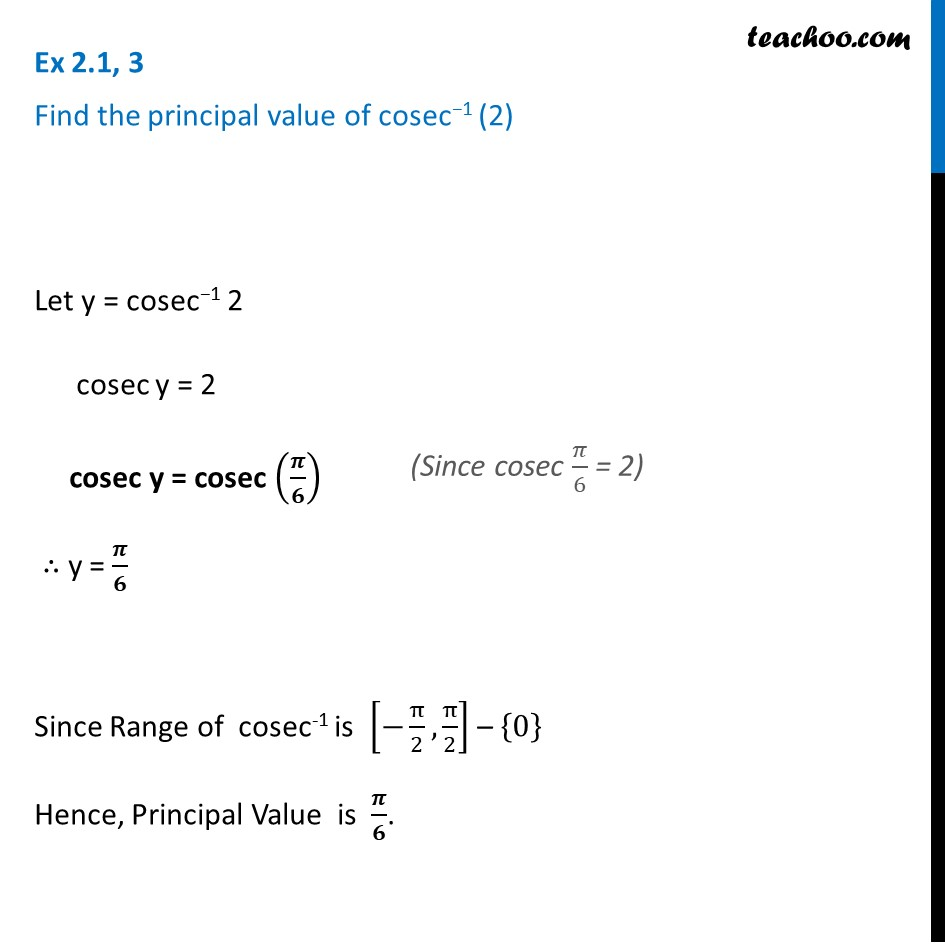1. Chapter 2 Class 12 Inverse Trigonometric Functions (Term 1)
2. Serial order wise
3. Ex 2.1

Transcript

Ex 2.1, 3 Find the principal value of cosec−1 (2) Let y = cosec−1 2 cosec y = 2 cosec y = cosec (𝝅/𝟔) ∴ y = 𝝅/𝟔 Since Range of cosec-1 is [−π/2,π/2] – {0} Hence, Principal Value is 𝝅/𝟔.

Ex 2.1

Chapter 2 Class 12 Inverse Trigonometric Functions (Term 1)
Serial order wise